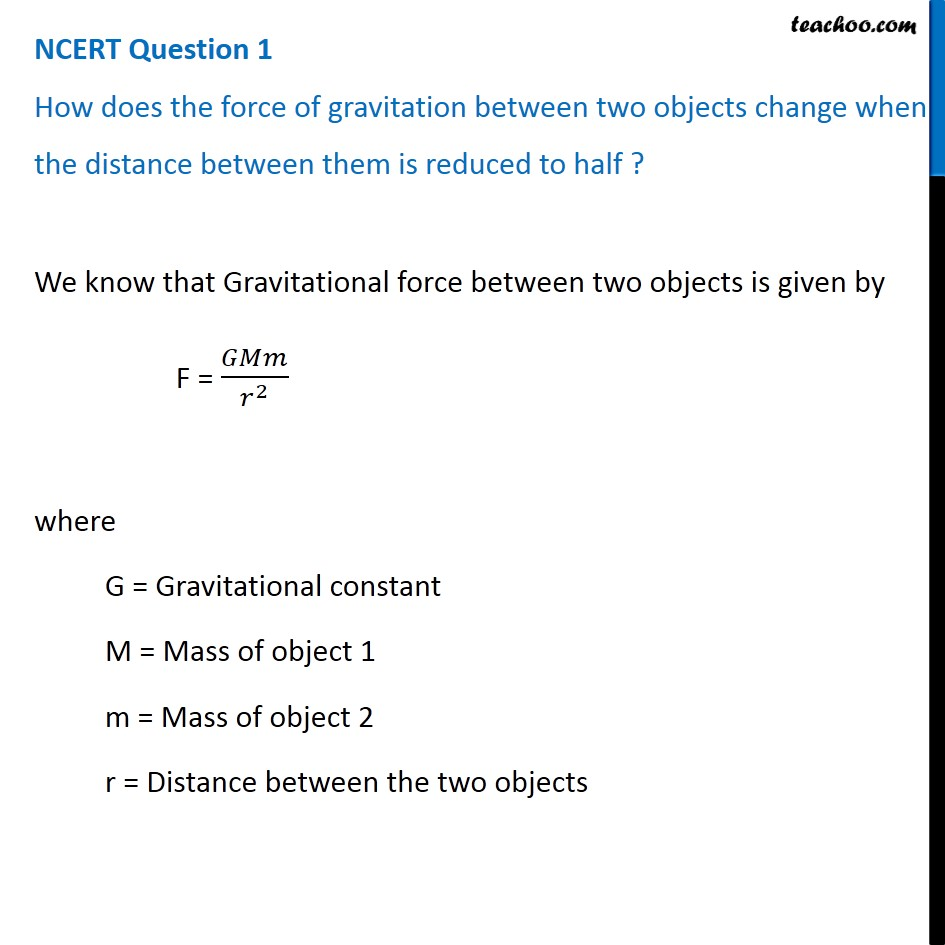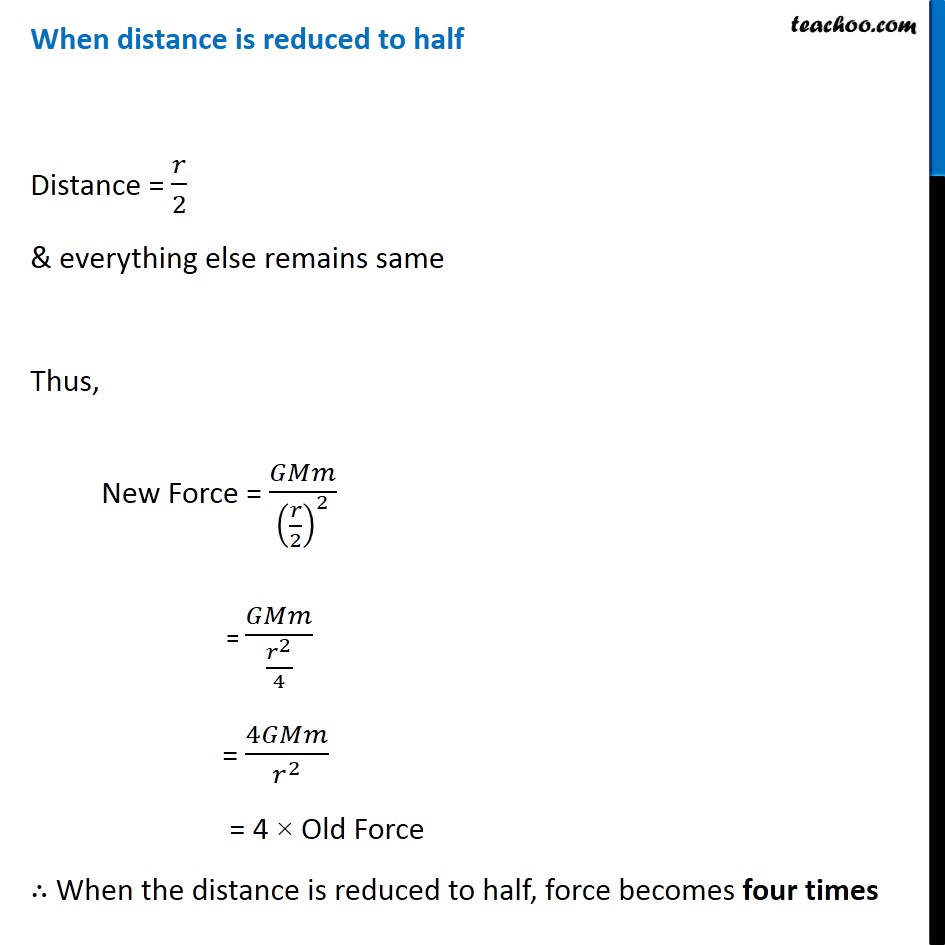NCERT Questions

Class 9
Chapter 10 Class 9 - GravitationLearn in your speed, with individual attention - Teachoo Maths 1-on-1 Class

### Transcript

NCERT Question 1 How does the force of gravitation between two objects change when the distance between them is reduced to half ? We know that Gravitational force between two objects is given by F = 𝐺𝑀𝑚/𝑟^2 where G = Gravitational constant M = Mass of object 1 m = Mass of object 2 r = Distance between the two objects When distance is reduced to half Distance = 𝑟/2 & everything else remains same Thus, New Force = 𝐺𝑀𝑚/(𝑟/2)^2 = 𝐺𝑀𝑚/(𝑟^2/4) = 4𝐺𝑀𝑚/𝑟^2 = 4 × Old Force ∴ When the distance is reduced to half, force becomes four times